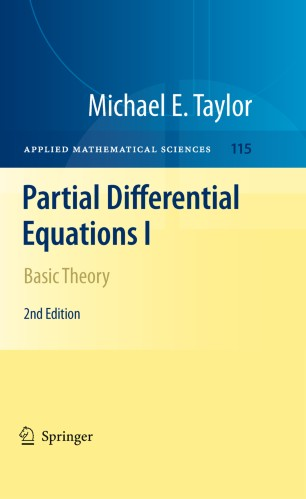# Partial Differential Equations I

## Basic Theory

• Michael E. TaylorTextbook

Part of the Applied Mathematical Sciences book series (AMS, volume 115)

1. Front Matter
Pages i-xxii
2. Michael E. Taylor
Pages 1-126
3. Michael E. Taylor
Pages 127-195
4. Michael E. Taylor
Pages 197-313
5. Michael E. Taylor
Pages 315-352
6. Michael E. Taylor
Pages 353-480
7. Michael E. Taylor
Pages 481-547
8. Michael E. Taylor
Pages 549-615
9. Michael E. Taylor
Pages 617-648
10. Back Matter
Pages 649-654

### Introduction

The first of three volumes on partial differential equations, this one introduces basic examples arising in continuum mechanics, electromagnetism, complex analysis and other areas, and develops a number of tools for their solution, in particular Fourier analysis, distribution theory, and Sobolev spaces. These tools are then applied to the treatment of basic problems in linear PDE, including the Laplace equation, heat equation, and wave equation, as well as more general elliptic, parabolic, and hyperbolic equations. The book is targeted at graduate students in mathematics and at professional mathematicians with an interest in partial differential equations, mathematical physics, differential geometry, harmonic analysis, and complex analysis.

In this second edition, there are seven new sections including Sobolev spaces on rough domains, boundary layer phenomena for the heat equation, the space of pseudodifferential operators of harmonic oscillator type, and an index formula for elliptic systems of such operators. In addition, several other sections have been substantially rewritten, and numerous others polished to reflect insights obtained through the use of these books over time.

Michael E. Taylor is a Professor of Mathematics at the University of North Carolina, Chapel Hill, NC.

Review of first edition: “These volumes will be read by several generations of readers eager to learn the modern theory of partial differential equations of mathematical physics and the analysis in which this theory is rooted.”

(SIAM Review, June 1998)

### Keywords

Basic Theory of ODE and Vector Fields Fourier Analysis Laplace Equation Linear Elliptic Equations Wave Equation

#### Authors and affiliations

• Michael E. Taylor
• 1
1. 1.Department of MathematicsUniversity of North CarolinaChapel HillUSA